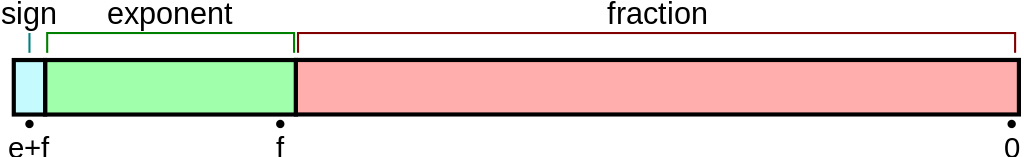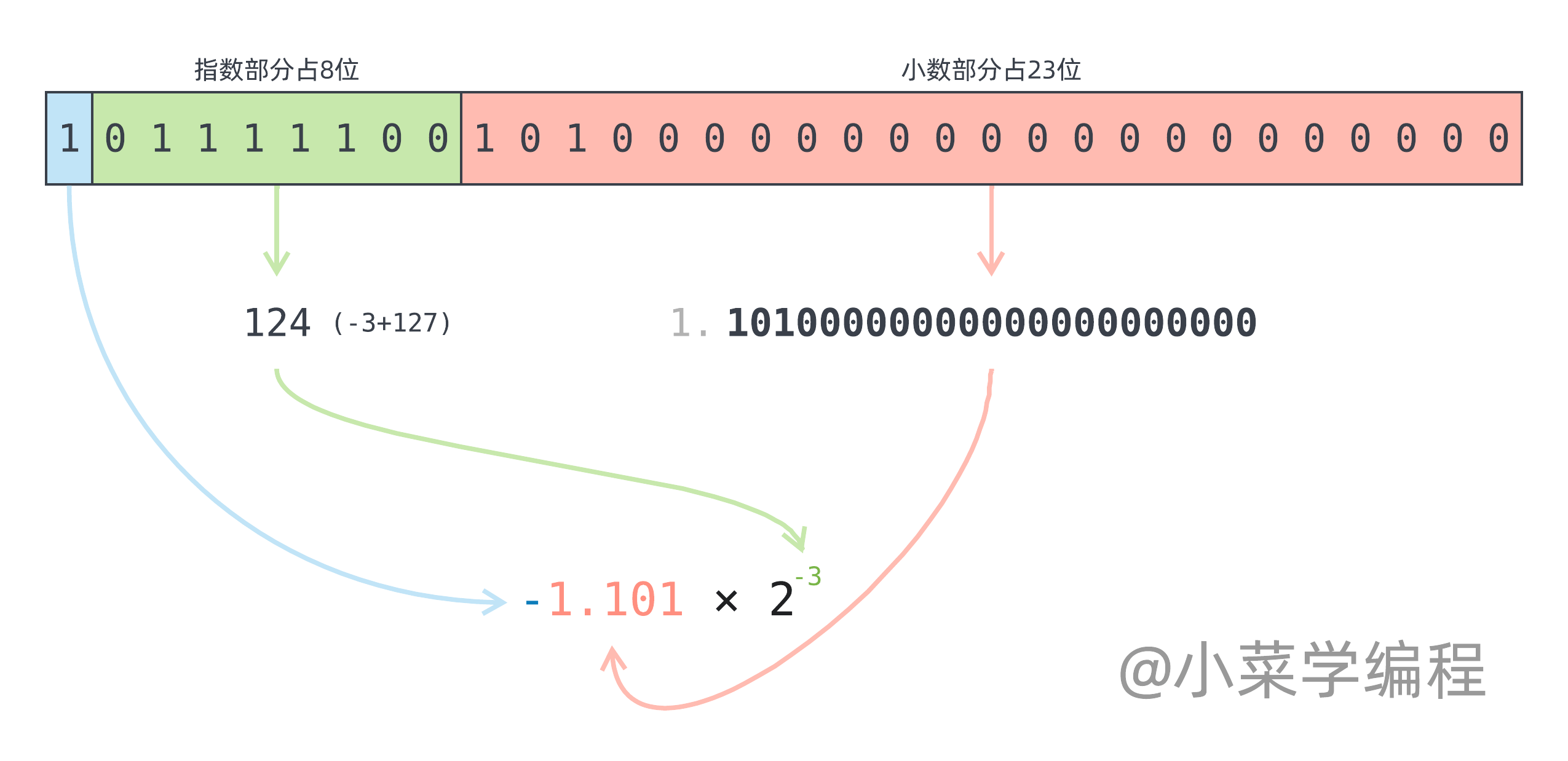# IEEE-754浮点数那些坑：0.1加0.2不等于0.3！

 1  0.1 + 0.2 === 0.3 // false 

0.1 加上 0.2 居然不等于 0.3 ，那结果等于多少呢？

 1  0.1 + 0.2 // 0.30000000000000004 

## 科学计数法

• n 必须是一个整数；
• $1 \le \left|a\right| \lt 10$ ，如果原数绝对值小于 1 或 大于等于 10 ，都可以通过改变 n 次幂来表示；

2 $2\times10^0$
200 $2\times10^2$
520 $5.2\times10^2$
-2021 $-2.021\times10^3$
2021.0402 $2.0210402\times10^3$
0.000020210402 $2.0210402\times10^{-5}$

## IEEE 754

### 二进制浮点数

$8+2+1+0.25+0.0625 = 11.3125$

### 二进制科学计数法

$1.0110101 \times 2^3$

IEEE 754 正是用这种方式来表示浮点数的！一个以科学计数法表示的浮点数，可以分为三个部分：

• 符号sign ），表示浮点数的正负，通常以 0 表示正，1 表示负；
• 小数fraction ），即例子中的 1.0110101 ，也叫作 有效数significand ）；
• 指数exponent ），表示 2n 次幂，即例子中的 3

### 封装细节

IEEE 754 将这三部分封装到一组二进制位中，位数分为 32 位和 64 位两种不同版本。位数越多，可以表示的数值范围越大，精度越高。主流编程语言都使用 64 位版本，但我们以更简单的 32 位版本来讲解，原理都是一样的。IEEE 754 32 位浮点数又称为单精度浮点数，由 32 个二进制位构成，其中：

• 符号sign ）占一位，第 31 位，0 表示正数，1 表示负数；
• 指数exponent ）占 8 位，第 23~30 位；
• 小数fraction ）占 23 位，第 0~22 位；

• 第一个可用数值为 1 ，表示 -126-126+127=1 ）；
• 最后一个可用数值为 254 ，表示 127127+127=254 ）；• 符号位决定符号，值为 1 说明这是一个负数；
• 指数部分数值为 124 ，实际指数需要再减去 127 ，得到：-3
• 小数部分需要加上固定个位（ 1 ），得到：1.10100000000000000000000

## 精度问题

$1.10011001100110011001101 \times 2^{-4} = 0.000110011001100110011001101$

0.000110011001100110011001101 0.100000001490116119384765625 32位浮点
0.000110011001100110011001100 0.0999999940395355224609375 最后一位改成零就偏小
0.0001100110011001100110011001 0.0999999977648258209228515625 再加一个一还是偏小
0.00011001100110011001100110011 0.09999999962747097015380859375 再加一个还是偏小
0.000110011001100110011001100111 0.1000000005587935447692871094 再加一个却偏大

 1 2  // 这个例子是64位版本浮点数，误差比32位版本要小一些 0.1 + 0.1 + 0.1 // 0.30000000000000004 

## 解决方案

Python 使用 decimal.Decimal 计算二进制小数 0.000110011001100110011001101 的值为例：

 1 2 3 4 5 6 7  >>> from decimal import Decimal >>> sum([ Decimal(2)**-i for i, v in enumerate('0000110011001100110011001101') if v == '1' ], Decimal(0)) 0.100000001490116119384765625 

## 附录

  1 2 3 4 5 6 7 8 9 10 11 12 13 14 15 16 17 18 19 20 21 22 23 24 25 26 27 28 29 30 31 32 33 34 35 36 37 38 39 40 41 42 43 44 45 46 47 48 49 50 51 52 53 54 55 56 57 58 59 60 61 62 63 64 65 66 67 68 69 70 71 72  #include void print_bits(unsigned long long value, int n) { for (int i=0; i0 && i%8==0) { printf("-"); } printf("%lld", (value>>(n-1-i)) & 1); } } void print_ieee754_layout(void *c, int bits) { unsigned long long value; int fracbits, expbits; if (bits == 32) { value = (unsigned long long)(*((unsigned int *)c)); // 32位浮点数，小数部分为23位，指数为8位，最高位是符号位 fracbits = 23; expbits = 8; } else { value = *((unsigned long long *)c); // 64位浮点数，小数部分为52位，指数为11位，最高位是符号位 fracbits = 52; expbits = 11; } // 取数掩码 unsigned long long fracmask = (1 << fracbits) - 1, expmask = (1 << expbits) - 1; // 分别取出各个部分 unsigned int sign = (value >> (bits-1)) & 1; unsigned long long exp = (value >> fracbits) & expmask; unsigned long long frac = value & fracmask; // 分别打印 printf("s=%u/%c", sign, sign == 1 ? '-' : '+'); printf(" exp="); print_bits(exp, expbits); printf("/%03llu", exp); printf(" frac="); print_bits(frac, fracbits); } void print_float(float f) { printf("% 20.9f: ", f); print_ieee754_layout(&f, 32); printf("\n"); } void print_double(double d) { printf("% 20.9f: ", d); print_ieee754_layout(&d, 64); printf("\n"); } int main() { print_float(0.1); print_float(0.2); print_float(-0.203125); print_double(0.1); print_double(0.2); print_double(-0.203125); return 0; }【随笔】系列文章首发于公众号【小菜学编程】，敬请关注：